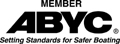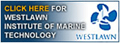Disclaimer:   I am not a spokesperson for the US Coast Guard or ABYC. For an official interpretation of regulations or standards you must contact the US Coast Guard or other organization referenced..   More.....

## Building A Sailing Pram

Building A 7 ft. 10 In. Sailing Pram (or Picnic Table Boat Building) Page 3

Calculating Capacity and flotation:

Finding Displacement Weight:

Float Plane. The float plane is that level in the boat at which water would come in if you added enough weight. If this were a rowing dinghy that point would be the lowest point of the gunwale. However, as a sailing dinghy, the lowest point is the top of the centerboard trunk, which is 3 or 4 inches below the gunwale. So the volume below the float plane is the volume that determines the capacity and the amount of flotation for this boat.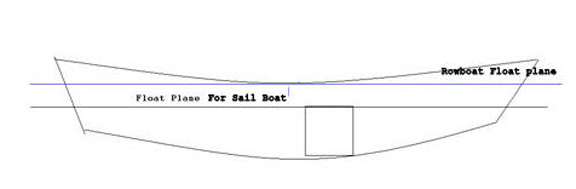This lowers the capacity to less than it would be if this was a rowing dinghy but considering that a sail boat heels far more than a rowboat, is a reasonable reduction in capacity for safety.

The capacity is based on the volume below this plane. The volume is multiplied by 62.4 lb. per cubic foot. To determine the displacement weight.

For manually propelled boats:
Maximum Weight Capacity = 3/10 of displacement weight
Maximum Persons Capacity = 9/10 of Maximum Weight Capacity.

Measuring the Boat and Calculating Volumes:

Since this is basically a flat bottom boat, it can be divided up into some simple shapes to make it easier to calculate volume.  Consider the bow and transom area as separate boxes, then divided up the boat into four sections. Each section will be a four sided polygon that can be easily measured and the volume calculated because they can be treated as rectangles.  The bow and transom volumes are triangles. The volume of a triangle is 1/2 the width, times the height, times the depth, or 1/2 the volume of rectangular box of the same height, depth and width.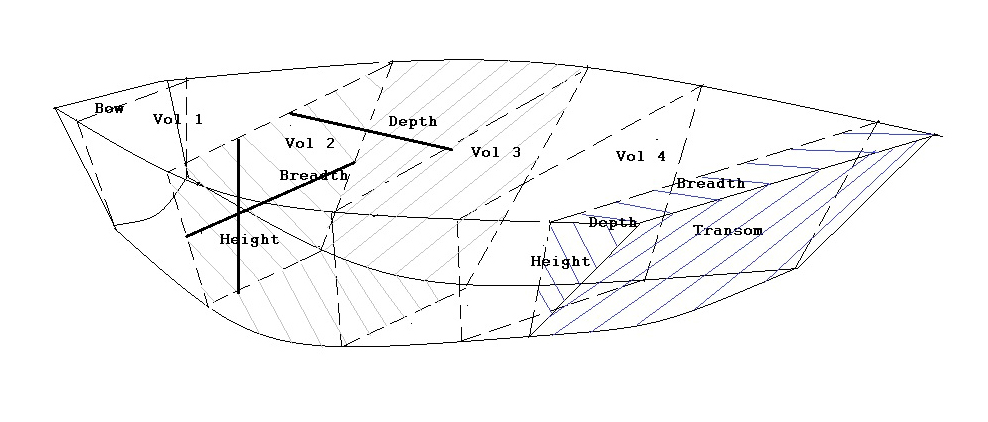The example, shown here (grey cross hatch), shows the height, breadth and depth of volume 2. The breadth used is the average breadth. The average is the top breadth plus the bottom breadth divided by two.

Calculate volume for the bow and the transom, vol. 1, 2, 3, and 4, and add them.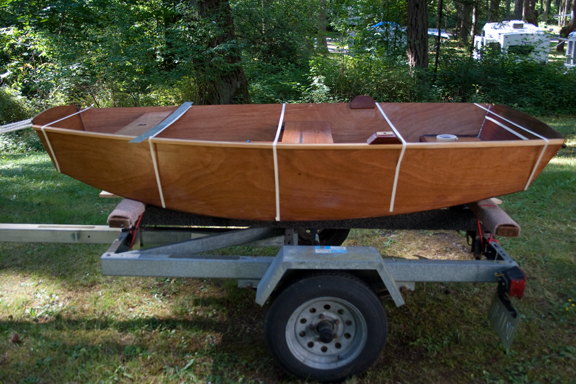The following photographs illustrate how to do this. Use some narrow tape to divide up the boat. I started at the bow, and marked off the triangular volume. I did the same for the transom. I then divided the length between the two ends by four. You can use more divisions. The smaller each space is the more accurate the final result will be. For a boat this size four is enough to get an accurate volume. The third photo shows the height of the centerboard trunk above the bottom of the boat, which is the lowest point at which water will come in. The static float plane has to be level with this height. The middle photo shows a line of tape running around the inside of the boat at this height.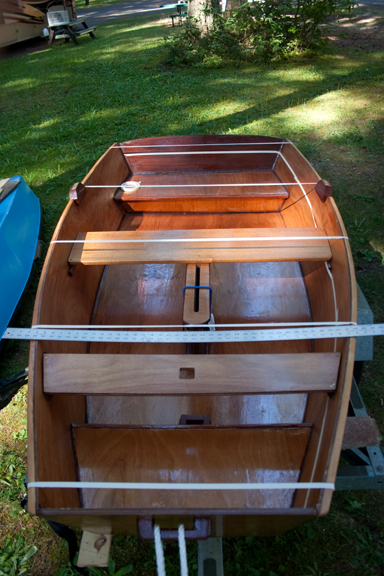If you measure the inside of the boat you have to add the thickness of the planking times two (two sides), for the width of the boat, and 1/4 inch for heights above the bottom. If you measure the outside then do not add the thickness of the planking.

Two Examples:  The bow and volume number two.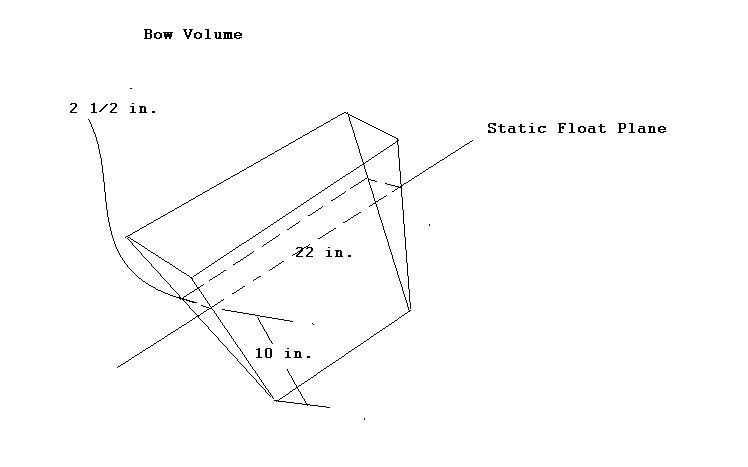The bow at the float plane is 23 1/4 inches wide, 10 inches high and 2 1/2 inches wide Bow Vol. 23 1/4  = 1.19375 ft.  10 in = 0.8333 ft.  2 1/2 in. = 0.2085 ft (1.9375 X 0.8333 X 0.2085)/2 = 0.1682 Cu. Ft.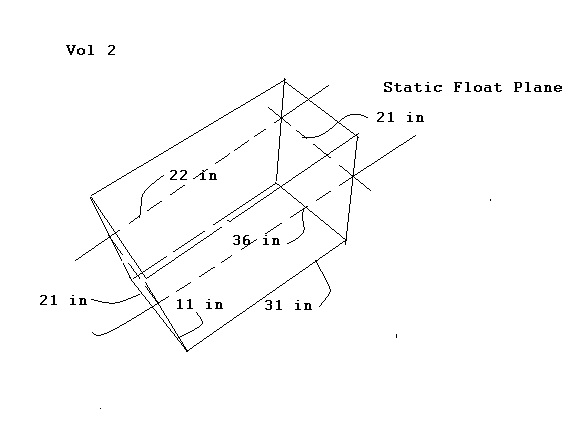Volume number two front width = 22 in.  Rear width = 36 in. Average width = 29.000 in = 2.4167 ft. Height = 10 in = 0.8333 ft. Depth = 21 in = 1.75 ft. 2.4167 X 0.8333 X 1.75 =  3.5242 Cu. ft.

Do the same operation for all the volumes.

On this boat the volume below the float plane equals 20.7018 Cu. Ft.

Displacement weight (weight of 20.7018 cu ft of fresh water)=20.7018 Cu. Ft. X 62.4 lb/cu ft

Displacement weight = 1291.792 lb.

There are other methods to calculate displacement weight:

1. Put weight in the boat until water comes in. Add up the weight and subtract the hull weight.

2. A variation on that, since most of us don't have 1000 lb. of weights laying around, is the bucket method. Use a bucket that is marked with the number of gallons it holds. Fill the bucket and pour it in the boat. Do this until the water is at the same level as the outside.  In other words, water flows in and out freely through the centerboard trunk. Count the number of buckets and multiply them times the gallons per bucket. Multiply that times 8 lb. per gallon (approximate weight of water is 8 lb.) That is the displacement weight.

Caution:  Do Not Do This With The Boat Out Of The Water. You Will break the boat!

Finding Maximum Capacities:

Maximum weight capacity = 3/10 of Displacement weight = 0.30 X 1291.792 = 387.5376 lb

Persons Capacity in pounds = 9/10 of Maximum Weight Capacity = 387.5736 X 9/10 = 348. 78

Persons = Maximum Weight Capacity + 32 divided by 141 = 348.78 + 32 / 141 = 2.7

I have done this using four decimal places but this is not necessary. You could round the displacement weight to 1292 and the Maximum weight capacity to 387.  The numbers would still be correct.

I then down rated the maximum persons pounds to 300. This allows me a capacity of 2.4, or in this case 2 people.  If you used the full number, 2.7 then it can be rounded to 3, but I feel 3 adults is too many for this little boat.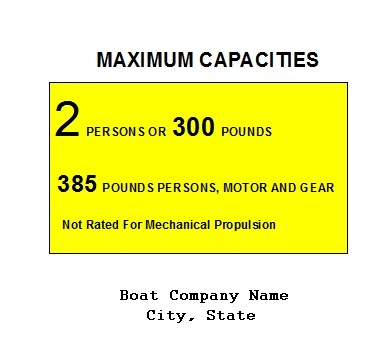So the Capacity Label reads as shown. It does not say US COAST GUARD MAXIMUM CAPACITIES because it is a sail boat. The capacity and flotation requirements do not apply to sailboats. So you also do not need the certification statement.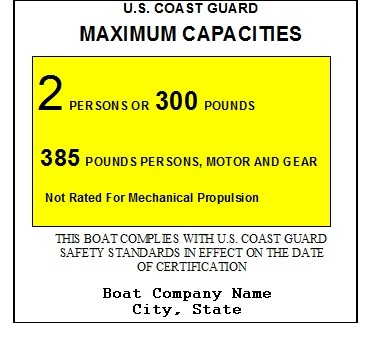However if I sold the same boat as a rowboat only, then the label would read as shown. This is because the capacity and flotation requirements apply to monohull manually propelled boats under 20 feet long.

Calculating Flotation

To determine the required amount of flotation you need to break down the weights into three categories.

Hull Weight

Persons Weight

Machinery Weight

This boat has no machinery weight.

Hull Weight = 60 lb. (weigh the boat)

Persons weight = 300 lb.

The Hull is wood so you multiply the hull weight by a buoyancy factor (see Table of Factors)

The factor for plywood = -0.81 ( the minus indicates the material is buoyant so adds buoyancy to the boat

Wb = boat weight in water.

Fb = the amount of flotation to float the boat weight.

B = buoyancy of the flotation material = 60.4

Wb = 60 lb X -0.81 = -48.6

Fb = -48.6 / 60.4 = -0.80 CU FT Since this is negative you do not need any additional flotation to float the boat.

PC = persons Capacity = 300 lb.

Fp = 1/2 X PC / b = 1/2 X 300 / 60.4 = 150 /60.4 = 1.44 Cu. ft.

You then add together the Flotation for the boat Fb, and the flotation for the persons Fp.

Ft = total flotation = 1.44 Cu. Ft. + (-0.80) cu. ft. = 0.64 cu. ft.

The bow seat is an air chamber of 0.58 cu. ft. and the rear seat is 0.88 cu. ft.

Total = 1.46 Cu. ft.   So we have over twice the required flotation.

If this were to be built without the centerboard trunk and sold as a rowing dinghy then the requirements would be greater.

Capacity For rowing dinghy:

The point where water comes in is now about three to four inches higher in the boat, so the boat has a larger displacement weight and can hold more weight. (the following are estimated from the measurements of the sailing dinghy)

Maximum Weight Capacity =  498 lbs

Maximum persons Capacity = 448 lbs

Maximum Person = 3

So by not having a centerboard we could add one more person safely.  However, from personal experience, I would be reluctant to put more than 2 persons in this boat.

Additionally, the Flotation requirements would be slightly greater but we still have more than required so no change.